Get instant live expert help with Excel or Google Sheets“My Excelchat expert helped me in less than 20 minutes, saving me what would have been 5 hours of work!”

#### Post your problem and you’ll get Expert help in seconds.

Your message must be at least 40 characters
Our professional Expert are available now. Your privacy is guaranteed.

# Learn How to Get Pivot Table Subtotals Grouped by Date

While working with Excel, we are able to create pivot tables and obtain specific data such as subtotals and grand totals by using the GETPIVOTDATA function.  This step by step tutorial will assist all levels of Excel users in getting pivot table subtotals grouped by date.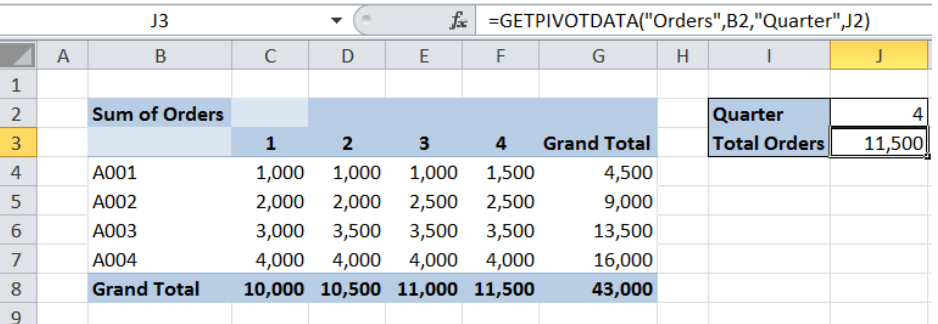Figure 1. Final result: Get pivot table data subtotals grouped by date

Final formula:  `=GETPIVOTDATA("Orders",B2,"Quarter",J2)`

## Syntax of the GETPIVOTDATA function

GETPIVOTDATA is used to retrieve specific data stored in a PivotTable report

`=GETPIVOTDATA(data_field, pivot_table, [field1, item1, field2, item2], ...)`

• data_field – The name of the data field, enclosed in quotation marks, which contains the data that we want to retrieve
• pivot_table  – reference to a cell or range of cells in a PivotTable report; determines the PivotTable report that contains the data that we want to retrieve
• field1, item1, field2, item2  – optional; pairs of field names and item names that determine the data that we want to retrieve.
• pairs can be in any order
• field names and items other than dates and numbers must be enclosed in quotation marks
• GETPIVOTDATA returns #REF! if pivot_table is not a range in the PivotTable report or if the arguments do not describe a visible field

## Setting up Our Data

Our source table contains three columns: Item (column B), Quarter (column C) and Orders (column D).  We will use this data to create a pivot table grouped by quarter.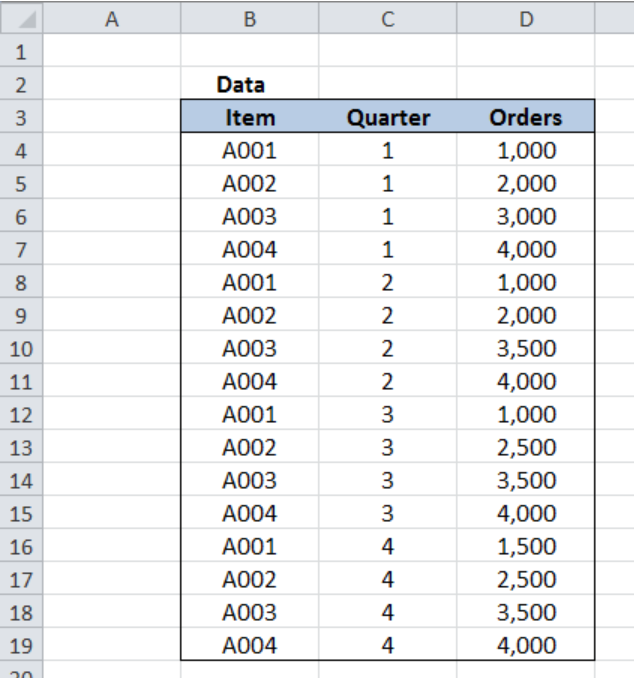Figure 2. Sample data to get pivot table data subtotals grouped by date

Below pivot table shows the subtotals and grand totals for orders per item and per quarter.  In cell J2, we enter the quarter whose subtotals we want to retrieve.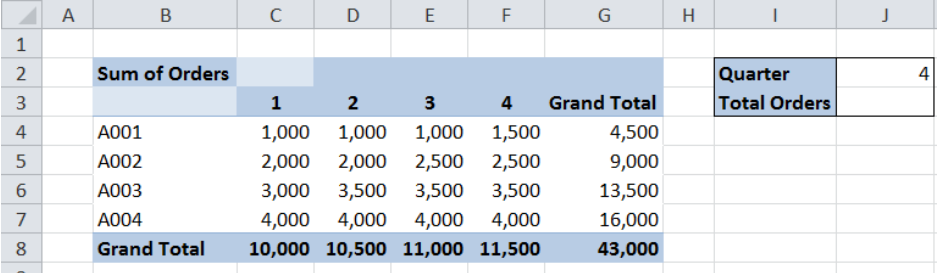Figure 3. Pivot table created from the source data

## Get pivot table subtotal for 4th quarter

We want to obtain the subtotal for orders in the 4th quarter.  We can easily retrieve data from a pivot table by using the GETPIVOTDATA function.  Let us follow these steps:

Step 1.  Select cell J3

Step 2.  Enter the formula: `=``GETPIVOTDATA("Orders",B2,"Quarter",J2)`

Step 3:  Press ENTER

Our data field is “Orders”.  The pivot_table reference is B2, which refers to the pivot table “Sum of Orders”.  The field/item pair refers to the field “Quarter”, and J2 contains the value 4, which means that we want to get the 4th quarter data.

The final result in cell J3 is 11,500, which is the subtotal for orders in the 4th quarter.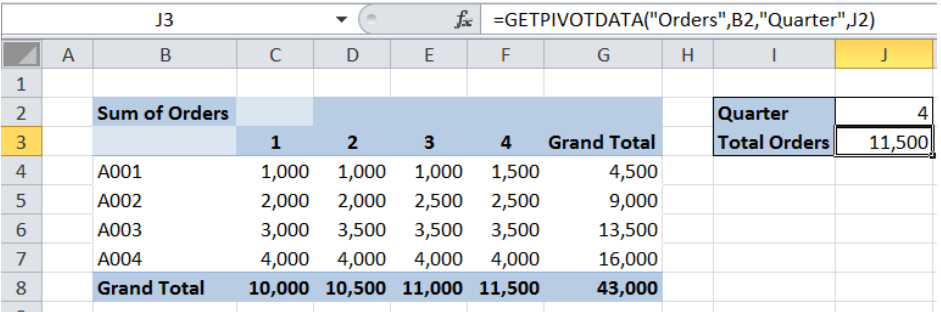Figure 4. Output: Get pivot table subtotal for 4th quarter

## Get subtotal for specific item

With the GETPIVOTDATA, we can retrieve more specific data by adding the appropriate field/item pairs in our formula.  For example, we want to obtain the subtotal for item A002 in the fourth quarter.

The formula in cell J3 becomes:

`=GETPIVOTDATA("Orders",B2,"Quarter",J2,"Item","A002")`

By adding the field/item pair “Item” and “A002” to our formula, we are able to obtain the specific data for A002 in the 4th quarter.  As a result, the value in cell J3 is 2,500.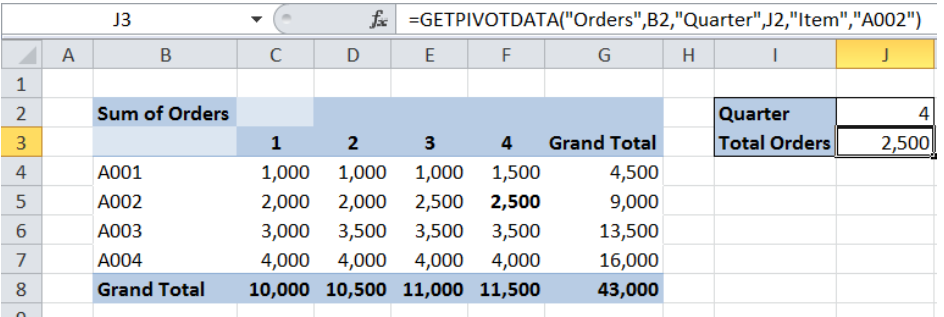Figure 5. Output: More specific subtotal for A002 in the 4th quarter

## Get pivot table grand total

In order to get the grand total, we simply omit the field/item pair in our formula.

The formula in cell J3 becomes:

`=GETPIVOTDATA("Orders",B2)`

The resulting grand total in cell J3 is 43,000.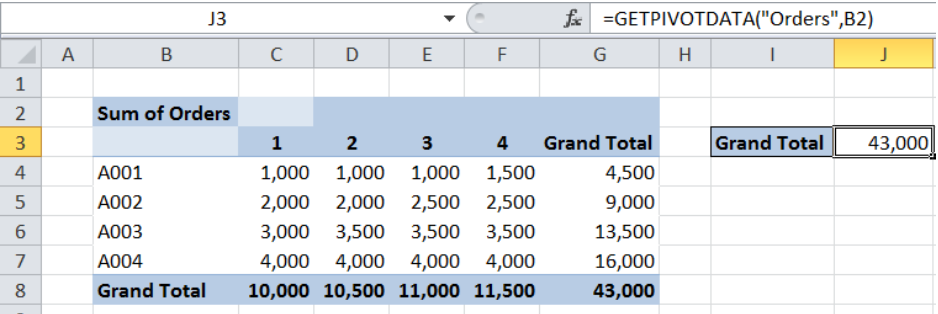Figure 6. Entering the GETPIVOTDATA formula to get grand total

Most of the time, the problem you will need to solve will be more complex than a simple application of a formula or function. If you want to save hours of research and frustration, try our live Excelchat service! Our Excel Experts are available 24/7 to answer any Excel question you may have. We guarantee a connection within 30 seconds and a customized solution within 20 minutes.

### Did this post not answer your question? Get a solution from connecting with the expert.Another blog reader asked this question today on Excelchat:## Subscribe to Excelchat.coAnother blog reader asked this question today on Excelchat: